# NCERT Solutions for Class 8 Maths Chapter 3 Exercise 3.1

NCERT Solutions for Class 8 Maths Chapter 3 Exercise 3.1 – Understanding Quadrilaterals, has been designed by the NCERT to test the knowledge of the student on the following topics :

• Introduction
• Polygons
Classification of polygons
Diagonals
Convex and concave polygons
Regular and irregular polygons
Angle sum property

### NCERT Solutions for Class 8 Maths Chapter 3 Exercise 3.1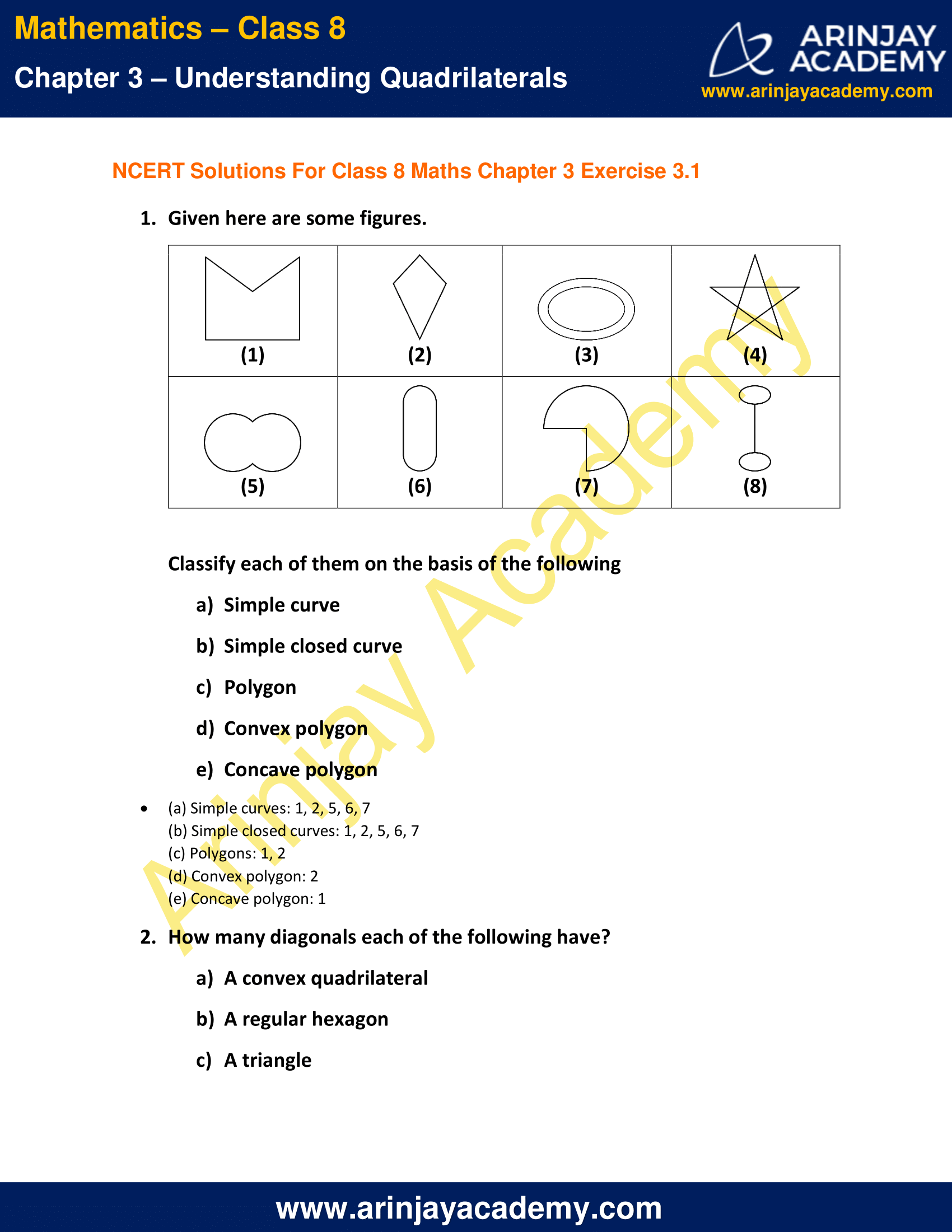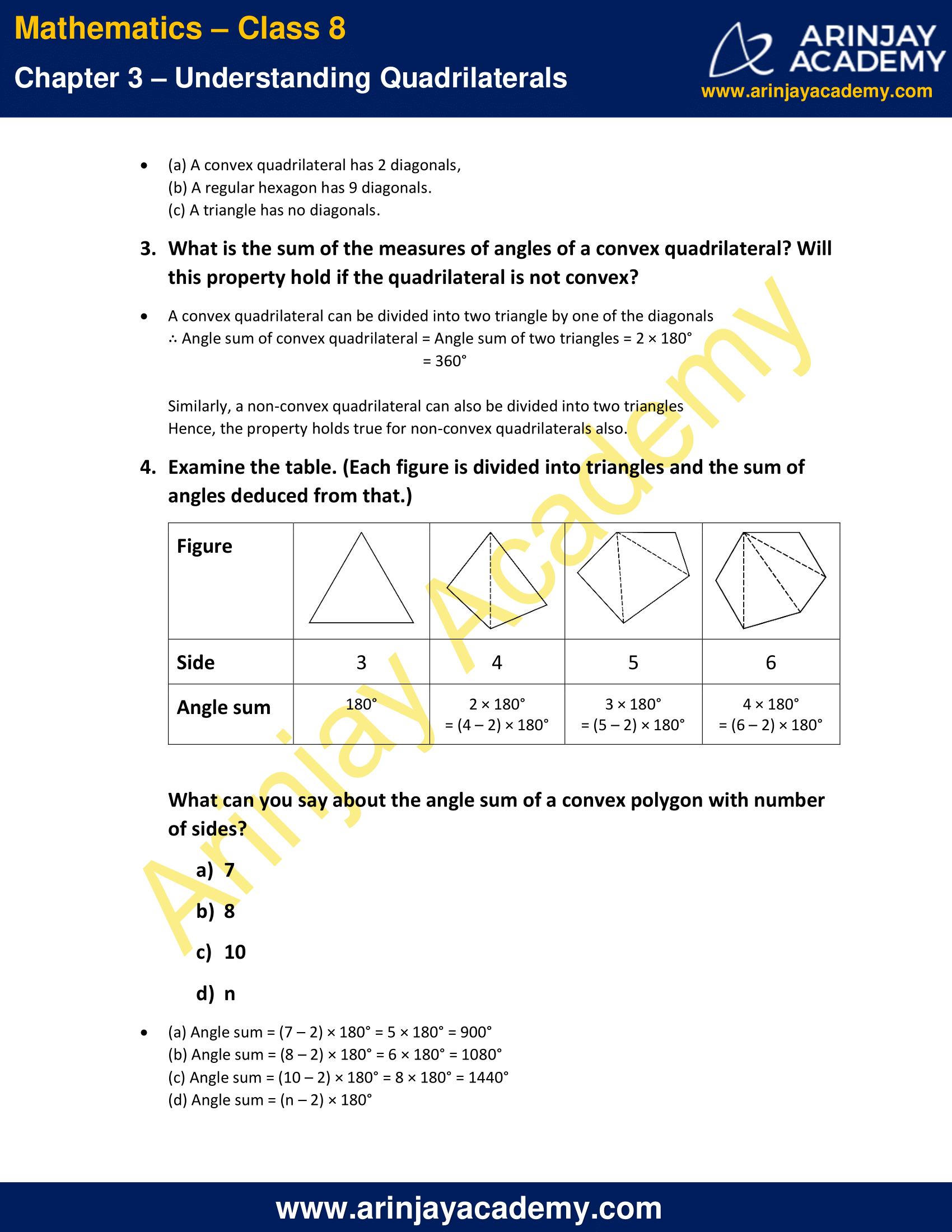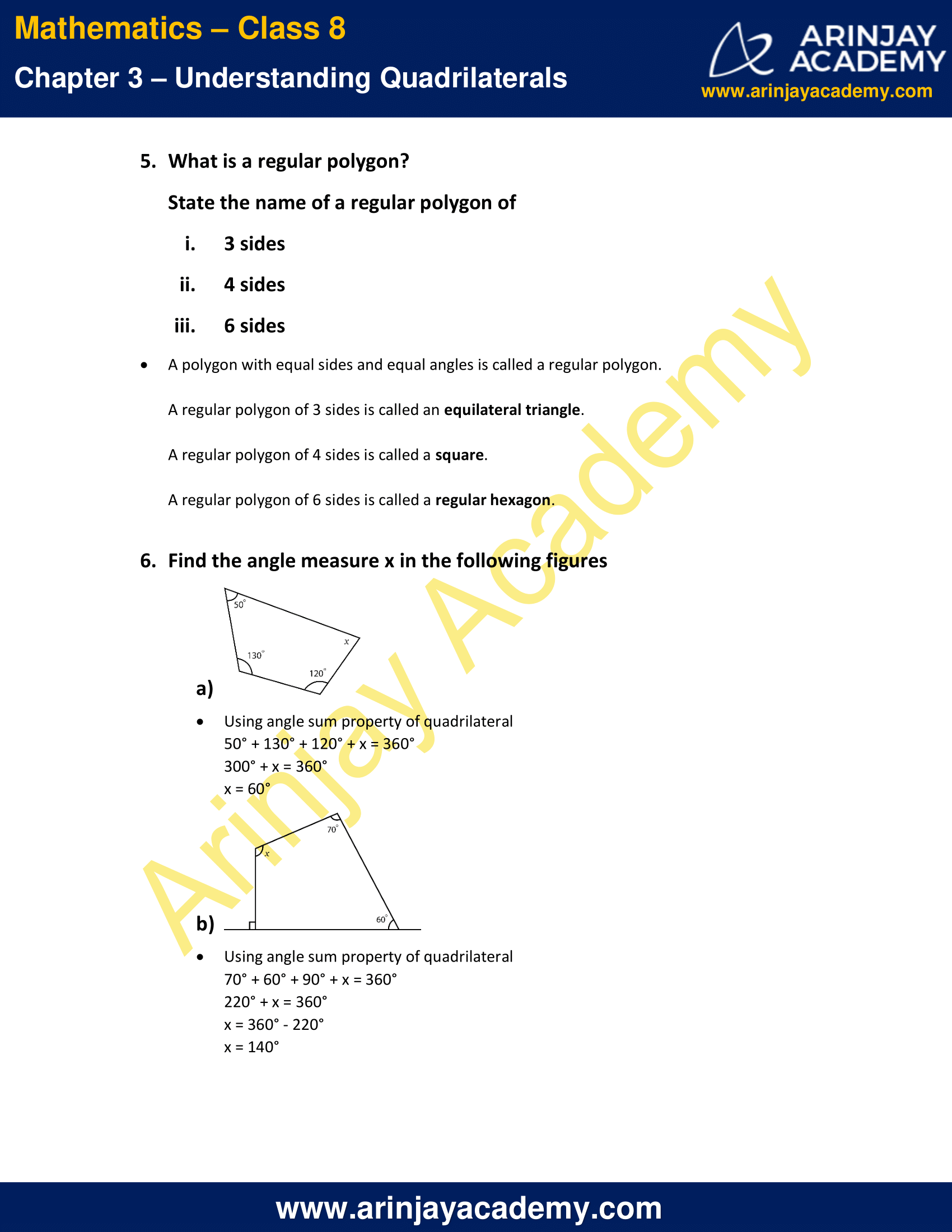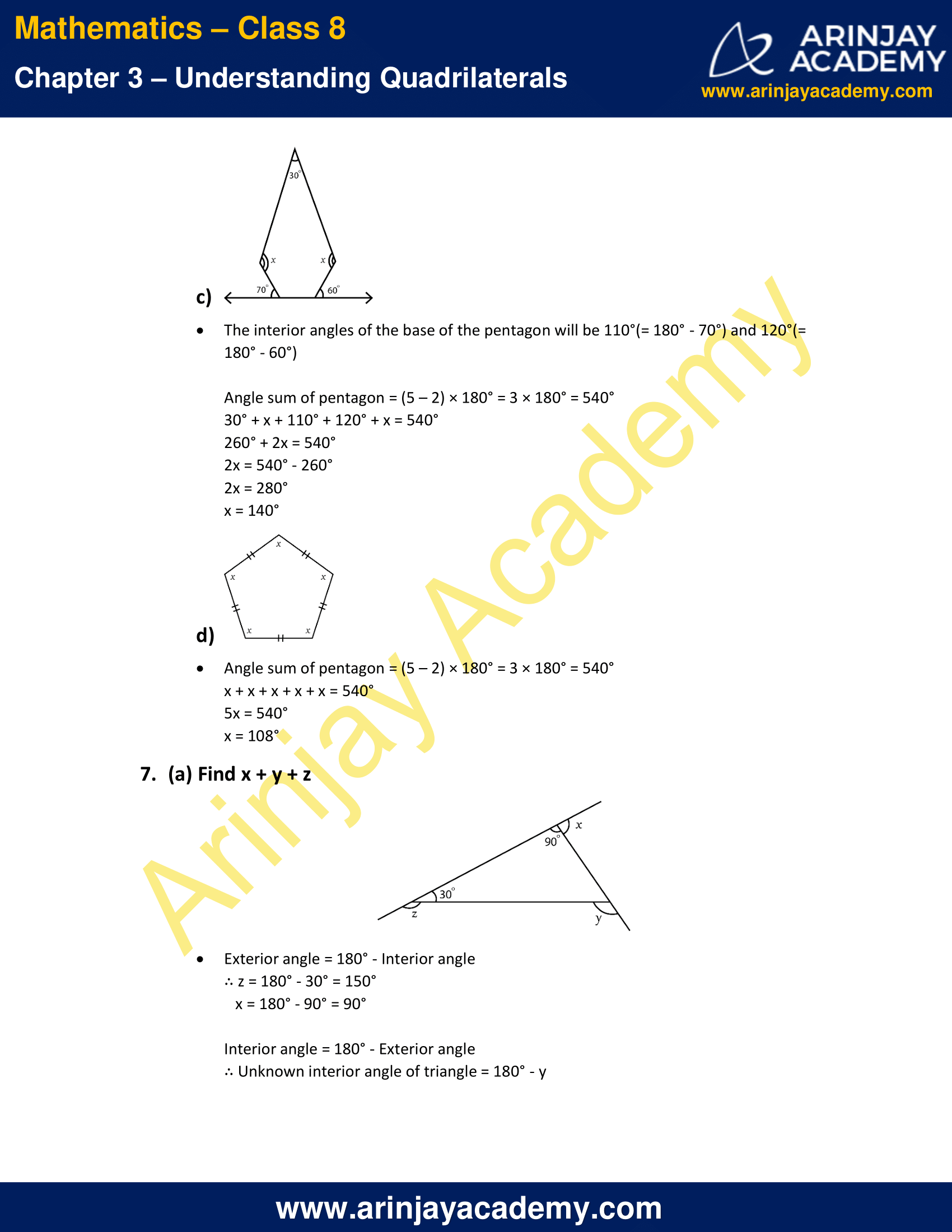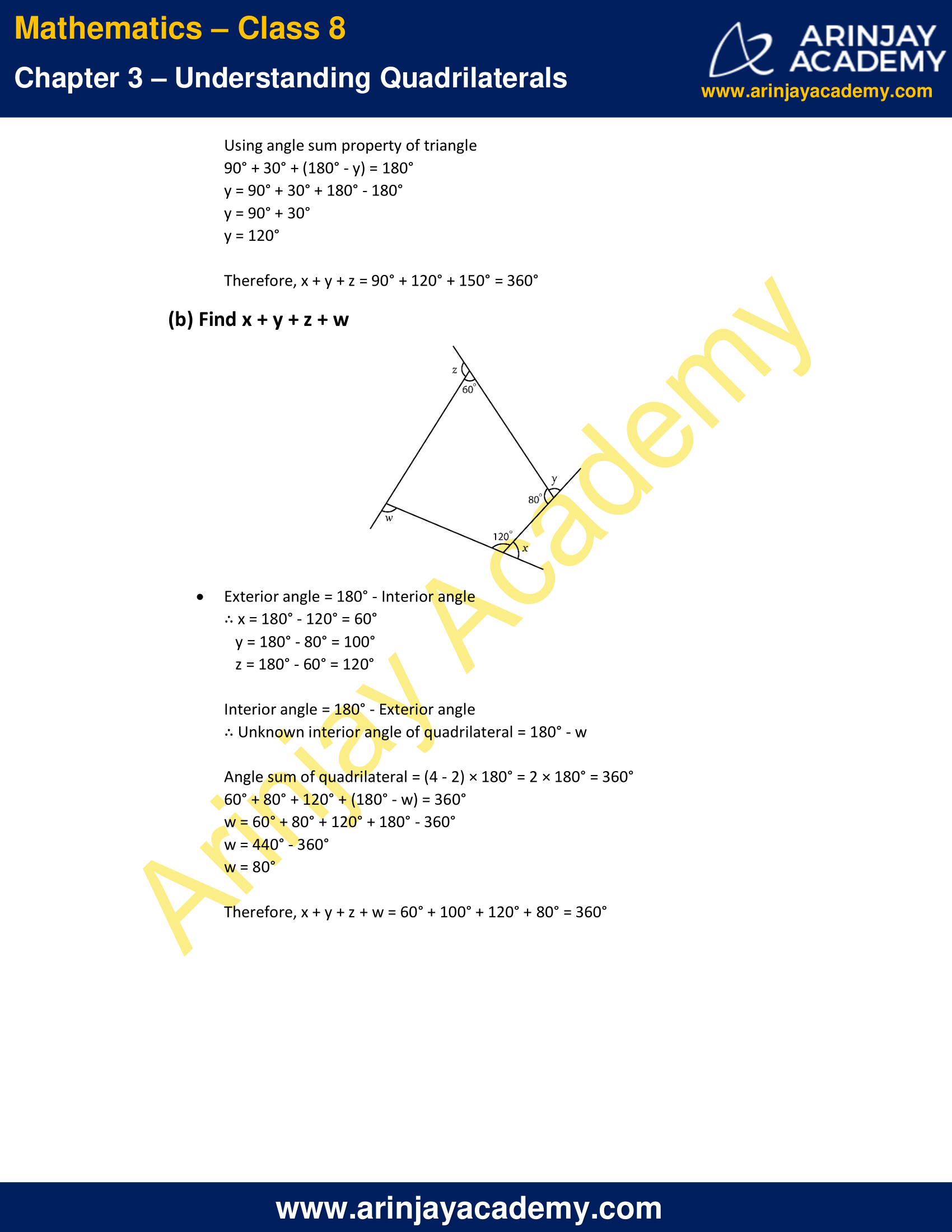NCERT Solutions for Class 8 Maths Chapter 3 Exercise 3.1

1. Given here are some figures.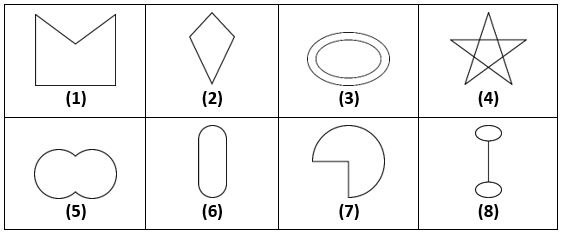Classify each of them on the basis of the following

a) Simple curve
b) Simple closed curve
c) Polygon
d) Convex polygon
e) Concave polygon

Solution:

(a) Simple curves: 1, 2, 5, 6, 7
(b) Simple closed curves: 1, 2, 5, 6, 7
(c) Polygons: 1, 2
(d) Convex polygon: 2
(e) Concave polygon: 1

2. How many diagonals each of the following have?

(b) A regular hexagon
(c) A triangle

Solution:

(a) A convex quadrilateral has 2 diagonals,
(b) A regular hexagon has 9 diagonals.
(c) A triangle has no diagonals.

3. What is the sum of the measures of angles of a convex quadrilateral? Will this property hold if the quadrilateral is not convex?

Solution:

A convex quadrilateral can be divided into two triangle by one of the diagonals
∴ Angle sum of convex quadrilateral
= Angle sum of two triangles
= 2 × 180°
= 360°
Similarly, a non-convex quadrilateral can also be divided into two triangles
Hence, the property holds true for non-convex quadrilaterals also.

4. Examine the table. (Each figure is divided into triangles and the sum of angles deduced from that.)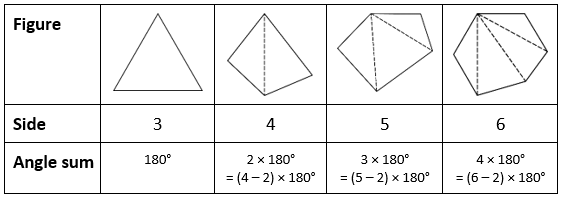What can you say about the angle sum of a convex polygon with number of sides?

(a) 7
(b) 8
(c) 10
(d) n

Solution:

(a) Angle sum = (7 – 2) × 180° = 5 × 180° = 900°
(b) Angle sum = (8 – 2) × 180° = 6 × 180° = 1080°
(c) Angle sum = (10 – 2) × 180° = 8 × 180° = 1440°
(d) Angle sum = (n – 2) × 180°

5. What is a regular polygon?
State the name of a regular polygon of

(i) 3 sides
(ii) 4 sides
(iii) 6 sides

Solution:

A polygon with equal sides and equal angles is called a regular polygon.

(i) A regular polygon of 3 sides is called an equilateral triangle.
(ii) A regular polygon of 4 sides is called a square.
(iii) A regular polygon of 6 sides is called a regular hexagon.

6. Find the angle measure x in the following figures

a)Solution:

Using angle sum property of quadrilateral
50° + 130° + 120° + x = 360°
300° + x = 360°
x = 60°

b)Solution:

Using angle sum property of quadrilateral
70° + 60° + 90° + x = 360°
220° + x = 360°
x = 360° – 220°
x = 140°

c)Solution:

The interior angles of the base of the pentagon will be 110°(= 180° – 70°) and 120°(= 180° – 60°)

Angle sum of pentagon = (5 – 2) × 180° = 3 × 180° = 540°
30° + x + 110° + 120° + x = 540°
260° + 2x = 540°
2x = 540° – 260°
2x = 280°
x = 140°

d)Solution:

Angle sum of pentagon = (5 – 2) × 180° = 3 × 180° = 540°

x + x + x + x + x = 540°
5x = 540°
x = 108°

7. (a) Find x + y + zSolution:

Exterior angle = 180° – Interior angle
∴ z = 180° – 30° = 150°
x = 180° – 90° = 90°

Interior angle = 180° – Exterior angle
∴ Unknown interior angle of triangle = 180° – y

Using angle sum property of triangle
90° + 30° + (180° – y) = 180°
y = 90° + 30° + 180° – 180°
y = 90° + 30°
y = 120°
Therefore, x + y + z = 90° + 120° + 150° = 360°

(b) Find x + y + z + wSolution:

Exterior angle = 180° – Interior angle
∴ x = 180° – 120° = 60°
y = 180° – 80° = 100°
z = 180° – 60° = 120°

Interior angle = 180° – Exterior angle
∴ Unknown interior angle of quadrilateral = 180° – w

Angle sum of quadrilateral = (4 – 2) × 180° = 2 × 180° = 360°
60° + 80° + 120° + (180° – w) = 360°
w = 60° + 80° + 120° + 180° – 360°
w = 440° – 360°
w = 80°

Therefore, x + y + z + w = 60° + 100° + 120° + 80° = 360°

The next Exercise for NCERT Solutions for Class 8 Maths Chapter 3 Exercise 3.2 – Understanding Quadrilaterals can be accessed by clicking here